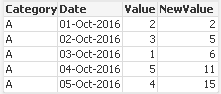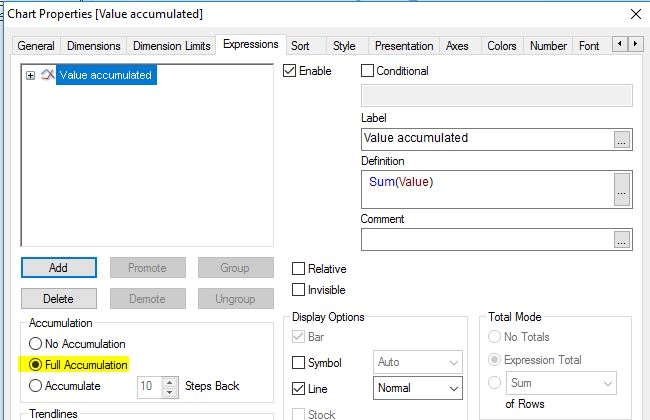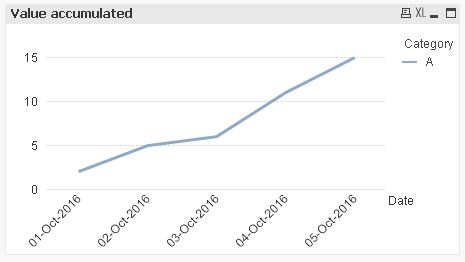New to QlikView

Discussion board where members can get started with QlikView.

Announcements
QlikWorld 2020: Join us May 11 - 14, 2020 in Phoenix, AZ. Register early and save \$400. Learn More
Highlighted
Contributor III

Plotting consecutive sum values in chart

CategoryDate

Value

A01-Oct-2016

2

A02-Oct-20163
A03-Oct-20161
A04-Oct-2016

5

A05-Oct-20164

The above sample data should be plotted in a Line Chart in way values sum up for each day. More like a Fibonacci series.

This should be my resultant table data

CategoryDate

Value

NewValue

A01-Oct-2016

2

2
A02-Oct-201635
A03-Oct-201616
A04-Oct-2016

5

11
A05-Oct-2016415

Is there a way to do this? Solutions for both at the script level or front end level (Using Expressions) would do the need.

Tags (2)
4 Replies
Valued Contributor II

Re: Plotting consecutive sum values in chartMVP

Re: Plotting consecutive sum values in chart

Perhaps thisUsing Straight Table

Take Dimension --> Category, Date

Expressions are

1) Sum(Value)

2) Rangesum(Below(Sum(Value),0),Above(Value,1,4))

And Second Expression, If you have more Category, Dates then for safety you can give Value like below

Rangesum(Below(Sum(Value),0),Above(Value,1,1000))

For Ref, PFA

Before develop something, think If placed (The Right information | To the right people | At the Right time | In the Right place | With the Right context)MVP

Re: Plotting consecutive sum values in chart

How long does accumulation restarts at the end of the month of end of the year? I am guessing it does restart for each category, right?MVP

Re: Plotting consecutive sum values in chart

Hi,

one front end solution could be the chart-in-built accumulation option:hope this helps

regards

Marco